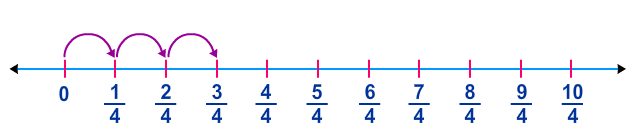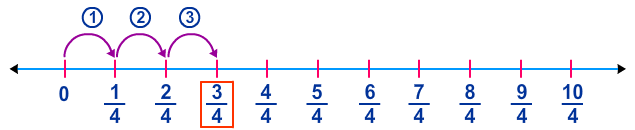# Multiply Unit Fractions by Whole Numbers (Using Number Lines)

Rate 0 stars Common Core
Quiz size:
Message preview:
Someone you know has shared quiz with you:

To play this quiz, click on the link below:

https://www.turtlediary.com/quiz/multiply-unit-fractions-by-whole-numbers-using-number-lines.html

Hope you have a good experience with this site and recommend to your friends too.

Login to rate activities and track progress.
Login to rate activities and track progress.

Consider the number line:Also, consider the multiplication sentence:

3 × ? =
 3 4

Let's identify the missing number to describe the number line.

Observe that on the given number line, starting from 0, three jumps of
1
4
3
4
.So, the number line represents the multiplication sentence:

3 ×
 1 4
=
 3 4
So, the missing number is
 1 4
.

ds

A B C D E F G H I J K L M N O P Q R S T U V W X Y Z

### Help

##### Remember :

The smallest number is the one that comes first while counting.

##### Solution :

To arrange the given numbers in order from smallest to greatest, find the smallest number among all the given numbers.

21,27,23

21 is the smallest number.